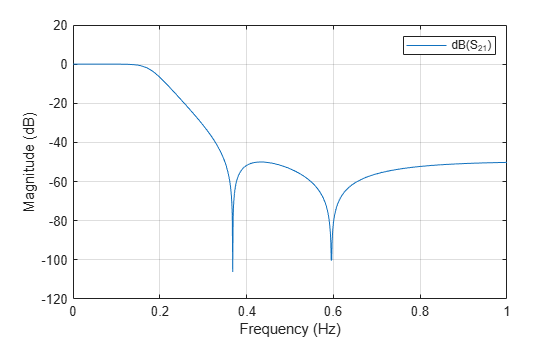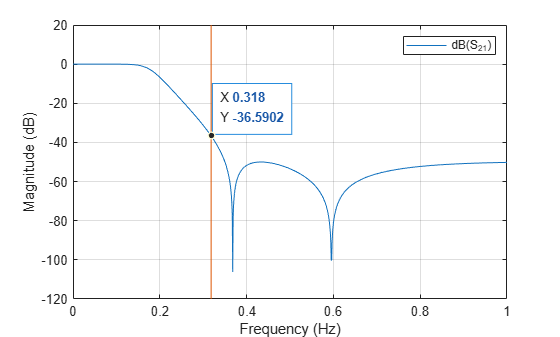Main Content

# Design, Visualize and Explore Inverse Chebyshev filter - I

This example shows how to determine the transfer function for a fifth-order inverse Chebyshev low-pass filter with 1 dB passband attenuation, cutoff frequency of 1 rad/sec, and a minimum attenuation of 50 dB in the stopband. Determine the amplitude response at 2 rad/sec .

The `rffilter object` is used to design a RF Filter. A filter requires a minimum set of parameters for it to be completely defined. Refer to the table in the `rffilter` documentation page which reflects this set of required parameters. Each set of parameters result in its corresponding syntax. Input these parameters as name-value pairs to `rffilter` to design the specified filter. Note that the parameters which are required but are not defined assume default values.

After initialization of an `rffilter` object, the property `DesignData` contains the complete solution of the filter designed. It is a structure which contains fields such as the computed factorized polynomials for the construction of the transfer function.

### Design Chebyshev Type II filter

```N = 5; % Filter order Fp = 1/(2*pi); % Passband cutoff frequency Ap = 1; % Passband attenuation As = 50; % Stopband attenuation```

Use `rffilter` object to create a desired filter. The only implementation type for Inverse Chebyshev is 'Transfer function'.

```r = rffilter('FilterType','InverseChebyshev','ResponseType','Lowpass', ... 'Implementation','Transfer function','FilterOrder',N, ... 'PassbandFrequency',Fp,'StopbandAttenuation',As, ... 'PassbandAttenuation',Ap);```

### Generate and visualize transfer function polynomial

Use `tf` function to generate transfer function polynomials.

```[numerator, denominator] = tf(r); format long g```

Display Numerator21 polynomial coefficients.

`disp('Numerator polynomial coefficients of Transfer function');`
```Numerator polynomial coefficients of Transfer function ```
`disp(numerator{2,1});`
``` Columns 1 through 3 0.0347736250821381 0 0.672768334081369 Columns 4 through 5 0 2.6032214373595 ```

Display Denominator polynomial coefficients.

`disp('Denominator polynomial coefficients of Transfer function');`
```Denominator polynomial coefficients of Transfer function ```
`disp(denominator);`
``` Columns 1 through 3 1 3.81150884154936 7.2631952221038 Columns 4 through 6 8.61344575257214 6.42982763112227 2.6032214373595 ```

Optionally, use Control System Toolbox to visualize all transfer functions.

`G_s = tf(numerator,denominator)`
```G_s = From input 1 to output... s^5 1: -------------------------------------------------------- s^5 + 3.812 s^4 + 7.263 s^3 + 8.613 s^2 + 6.43 s + 2.603 0.03477 s^4 + 0.6728 s^2 + 2.603 2: -------------------------------------------------------- s^5 + 3.812 s^4 + 7.263 s^3 + 8.613 s^2 + 6.43 s + 2.603 From input 2 to output... 0.03477 s^4 + 0.6728 s^2 + 2.603 1: -------------------------------------------------------- s^5 + 3.812 s^4 + 7.263 s^3 + 8.613 s^2 + 6.43 s + 2.603 s^5 2: -------------------------------------------------------- s^5 + 3.812 s^4 + 7.263 s^3 + 8.613 s^2 + 6.43 s + 2.603 Continuous-time transfer function. ```

### Visualize amplitude response of filter

```frequencies = linspace(0,1,1001); Sparam = sparameters(r, frequencies);```

Note: S-parameters computes the transfer function using quadratic (lowpass/highpass) or quartic (bandpass/bandstop) factorized forms. These factors are used to construct the polynomials. The polynomial form is numerically unstable for larger filter order so the preferred form is the factorized quadratic/quartic forms. These factorized parts are present in r.DesignData. For example, the numerator21 can be accessed using r.DesignData.Numerator21.

`l = rfplot(Sparam,2,1);`### Amplitude response of filter at specified frequency

```freq = 2/(2*pi); hold on; setrfplot('noengunits',false);```

Note: To use rfplot and plot on the same figure use setrfplot. Type 'help setrfplot' in command window for information.

```plot(freq*ones(1,101),linspace(-120,20,101)); setrfplot('engunits',false); [~,freq_index]= min(abs(frequencies-freq)); datatip(l,'DataIndex',freq_index);```Using the datatip, the magnitude at 2 rad/sec is found to be -36.59 dB.

Evaluate the exact value at 2 rad/sec.

```S_freq = sparameters(r,freq); As_freq = 20*log10(abs(rfparam(S_freq,2,1))); sprintf('Amplitude response at 2 rad/sec is %d dB',As_freq)```
```ans = 'Amplitude response at 2 rad/sec is -3.668925e+01 dB' ```

### Calculate stopband frequency at As

```Fs = r.DesignData.Auxiliary.Wx*r.PassbandFrequency; sprintf('Stopband frequency at -%d dB is: %d Hz',As, Fs)```
```ans = 'Stopband frequency at -50 dB is: 3.500241e-01 Hz' ```

 Ellis, Michael G. Electronic Filter Analysis and Synthesis. Boston: Artech House, 1994.

Download ebook